Science Questions

# 9th Std Science Lesson Wise Questions in English – Part 1

9th Std Science Lesson Wise Questions in English

9th Science Lesson 1 Questions in English

1] Measurement

1. Assertion (A): Length, mass, time are Fundamental quantities

Reason (R): Quantities which cannot be expressed in terms of any other physical quantities are called fundamental quantities

1. Both (A) and (R) are correct, but (R) does not explain (A)
2. Both (A) and (R) are wrong
3. Both (A) and (R) are correct and (R) explains (A)
4. (A) is Correct and (R) is wrong

Explanation

Physical quantity is a quantity that can be measured. Physical quantities can be classified into two: fundamental quantities and derived quantities. Quantities which cannot be expressed in terms of any other physical quantities are called fundamental quantities. Example: Length, mass, time, temperature etc. Quantities which can be expressed in terms of fundamental quantities are called derived quantities. Example: Area, volume, density etc.

2. Which of the following statement is correct?

1. A unit is a standard quantity with which the unknown quantities are compared
2. It is defined as a specific magnitude of a physical quantity that has been adopted by law or convention.
3. 1 alone
4. 2 alone
5. 1, 2
6. None

Explanation

A unit is a standard quantity with which the unknown quantities are compared. It is defined as a specific magnitude of a physical quantity that has been adopted by law or convention. For example, feet is the unit for measuring length. That means, 10 feet is equal to 10 times the definite pre-determined length, called feet.

3. When was SI system of units developed and recommended by General Conference on Weights and Measures?

1. 1991
2. 1960
3. 1981
4. 1935

Explanation

At the end of the Second World War there was a necessity to use worldwide system of measurement. Hence, SI (International System of Units) system of units was developed and recommended by General Conference on Weights and Measures at Paris in 1960 for international usage.

4. Which of the following statement is correct?

1. SI system of units is the modernised and improved form of the previous system of units
2. There are six fundamental units in the SI system of units
3. It is based on a certain set of fundamental units from which derived units are obtained by proper combination
4. 1, 2
5. 1, 3
6. 2, 3
7. All the above

Explanation

SI system of units is the modernised and improved form of the previous system of units. It is accepted in almost all the countries. It is based on a certain set of fundamental units from which derived units are obtained by proper combination. There are seven fundamental units in the SI system of units. The units used to measure the fundamental quantities are called fundamental units and the units which are used to measure the derived quantities are called derived units

5. Match the following

1. Energy 1. N
2. Surface tension 2. kgms–1
3. Momentum 3. N/m
4. Force 4. Nm
5. 1, 4, 2, 3
6. 4, 1, 2, 3
7. 4, 3, 2, 1
8. 3, 4, 1, 2

Explanation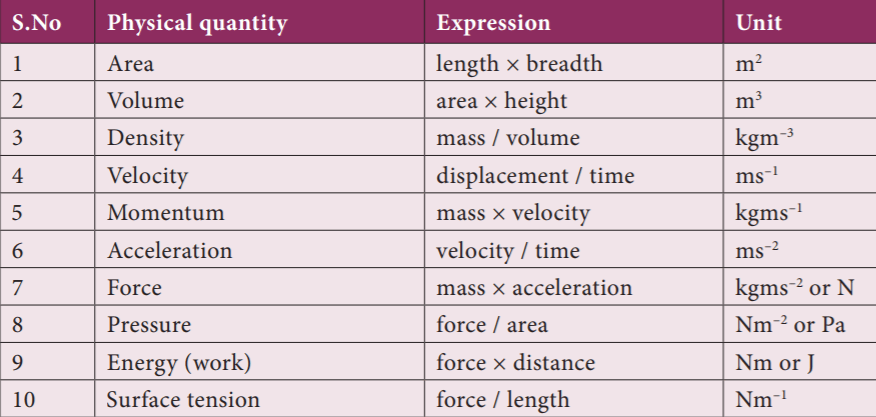6. Match the following

1. Luminous intensity 1. Mole
2. Length 2. Metre
3. Amount of substance 3. Candela
4. Electric current 4. Ampere
5. 1, 3, 2, 4
6. 3, 1, 4, 2
7. 2, 1, 4, 3
8. 3, 2, 1, 4

Explanation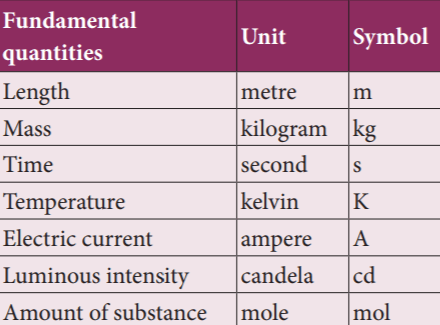7. Which of the following statement is correct?

1. Length is the extent of something between two points
2. The SI unit of length is metre
3. One metre is the distance travelled by light through vacuum in 1 second
4. 1, 2
5. 1, 3
6. 2, 3
7. All the above

Explanation

Length is the extent of something between two points. The SI unit of length is metre. One metre is the distance travelled by light through vacuum in 1/29,97,92,458 second.

8. Which of the following unit are used to measure very large distance?

1. Astronomical unit
2. Parsec
3. Light year
4. 1, 2
5. 1, 3
6. 2, 3
7. All the above

Explanation

In order to measure very large distance (distance of astronomical objects) we use the following units.

• Astronomical unit
• Light year
• Parsec

9. Match the following

1. Light year 1. 1.496 × 10^11 m
2. Parsec 2. 3.26 light year
3. Astronomical unit 3. 9.46 × 10^15 m
4. 3, 1, 2
5. 3, 2, 1
6. 2, 1, 3
7. 1, 3, 2

Explanation

Astronomical unit (AU): It is the mean distance of the centre of the Sun from the centre of the Earth. 1 AU = 1.496 × 10^11 m

Light year: It is the distance travelled by light in one year in vacuum and it is equal to 9.46 × 1015 m

Parsec: Parsec is the unit of distance used to measure astronomical objects outside the solar system. 1 Parsec = 3.26 light year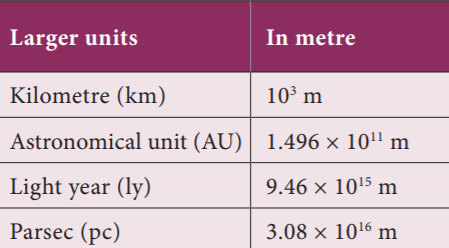10. What is the distance from the sun of Most of the stars visible to the unaided eye in the night sky?

1. 500 Parsec
2. 1500 Parsec
3. 50 Parsec
4. 5 Parsec

Explanation

The nearest star alpha centauri is about 1.34 parsec from the sun. Most of the stars visible to the unaided eye in the night sky are within 500 parsec distance from the sun.

11. Which of the following statement is correct?

1. To measure small distances such as distance between two atoms in a molecule, size of the nucleus and wavelength etc
2. we use multiples of ten for it.
3. These quantities are measured in Angstrom unit
4. 1, 2
5. 1, 3
6. 2, 3
7. All the above

Explanation

To measure small distances such as distance between two atoms in a molecule, size of the nucleus and wavelength etc. we use submultiples of ten. These quantities are measured in Angstrom unit.

12. Match the following

1. Micron 1. 10^–15 m
2. Nanometre 2. 10^–3 m
3. Centimetre 3. 10^–9 m
4. Fermi 4. 10^–6 m
5. 2, 3, 1, 4
6. 1, 2, 4, 3
7. 4, 3, 2, 1
8. 3, 1, 2, 4

Explanation13. Which of the following statement is correct?

1. Mass is the quantity of matter contained in a body
2. One kilogram is the mass of a particular international prototype cylinder made of platinum, kept at the International Bureau of Weights and Measures at Sevres, France
3. The SI unit of mass is kilogram
4. 1, 2
5. 1, 3
6. 2, 3
7. All the above

Explanation

Mass is the quantity of matter contained in a body. The SI unit of mass is kilogram (kg). One kilogram is the mass of a particular international prototype cylinder made of platinum-iridium alloy, kept at the International Bureau of Weights and Measures at Sevres, France. The unit gram (g) and milligram (mg) are the submultiples of ten (1/10) of the unit kg. Similarly, quintal and metric tonne are multiples of ten (× 10) of the unit kg.

14. Match the following

1. 1 g 1. 1000 kg
2. 1 mg 2. 0.001 kg
3. 1 quintal 3. 100 kg
4. 1 metric tonne 4. 0.000001 kg
5. 1, 2, 4, 3
6. 2, 1, 3, 4
7. 2, 4, 3, 1
8. 2, 1, 3, 4

Explanation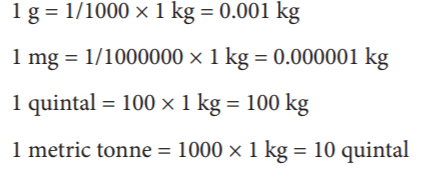15. 1 atomic mass unit is equal to_____

1. 1/12th of the mass of C12 atom
2. 1/12th of the mass of C11 atom
3. 1/12th of the mass of C14 atom
4. 1/12th of the mass of C13 atom

Explanation

Mass of a proton, neutron and electron can be determined using atomic mass unit (amu).

1 atomic mass unit = 1/12th of the mass of C12 atom

16. Mass of 1l of water = 1kg, Mass of 1 litre petrol=______

1. 10 kg
2. Same as that of water
3. Mass of the liquids vary with their density
4. None

Explanation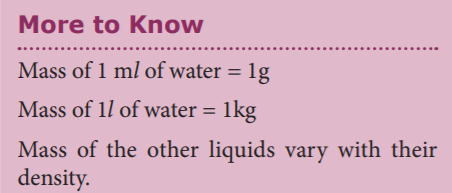17. Which of the following statement is correct?

1. Time is a measure of duration of events and the intervals between them.
2. The SI unit of time is second.
3. Larger units for measuring time are day, month, year and millennium
4. 1, 2
5. 1, 3
6. 2, 3
7. All the above

Explanation

Time is a measure of duration of events and the intervals between them. The SI unit of time is second. One second is the time required for the light to propagate 29,97,92,458 metres through vacuum. It is also defined as 1/86, 400th part of a mean solar day. Larger units for measuring time are day, month, year and millennium etc. 1 millenium = 3.16 × 10^9 s.

18. What is the SI unit of temperature?

1. Joule
2. Kelvin
3. degree Celsius
4. Fahrenheit

Explanation

Temperature is the measure of hotness or coldness of a body. SI unit of temperature is kelvin (K). The other units for measuring temperature are degree celsius (°C) and fahrenheit (F).

19. ________ is commonly known as absolute zero

1. Zero degree Celsius
2. Zero Fahrenheit
3. Zero kelvin
4. All the above

Explanation

One kelvin is the fraction (1/273.16) of the thermodynamic temperature of the triple point of water (The temperature at which saturated water vapour, pure water and melting ice are in equilibrium). Zero kelvin (0 K) is commonly known as absolute zero.

20. Which of the following statement is correct?

1. Unit prefixes are the symbols placed before the symbol of a unit to specify the order of magnitude of the quantity
2. They are useful to express very large and very small quantities
3. A unit prefix stands for a specific positive or negative power of 10.
4. 1, 2
5. 1, 3
6. 2, 3
7. All the above

Explanation

Unit prefixes are the symbols placed before the symbol of a unit to specify the order of magnitude of the quantity. They are useful to express very large and very small quantities. k (kilo) is the unit prefix in the unit, Kilo-meter. A unit prefix stands for a specific positive or negative power of 10.

21. Match the following

1. Peta 1. 10^6
2. Mega 2. 10^2
3. Deca 3. 10^15
4. Hector 4. 10^1
5. 1, 3, 2, 4
6. 2, 1, 3, 4
7. 3, 1, 4, 2
8. 1, 3, 2, 4

Explanation22. What is the mass of electron?

1. 9.11 × 10^-31 kg
2. 2.2 × 10^41 kg
3. 2.2 × 10^41 kg
4. 9.11 × 10^31 kg

Explanation

The physical quantities vary in different proportion like from 10^-15 m being the diameter of nucleus to 10^26 m being the distance between two stars and 9.11 × 10^-31 kg being the mass of electron to 2.2 × 10^41 kg being the mass of the milky way galaxy.

23. The smallest length which can be measured by metre scale is called________

1. Least count
2. Main count
3. Least error

Explanation

In our daily life, we use metre scale for measuring lengths. They are calibrated in cm and mm. The smallest length which can be measured by metre scale is called least count.

24. What accuracy can be made using Vernier caliper?

1. 0.01 mm
2. 0.01m
3. 0.1 mm
4. 0.1 cm

Explanation

Usually the least count of a scale is 1 mm. We can measure the length of objects upto 1 mm accuracy with this scale. By using vernier caliper we can have an accuracy of 0.1 mm and with screw gauge we can have an accuracy of 0.01 mm.

25. In which of the following scale of Vernier caliper is graduated?

1. mm
2. cm
3. m
4. fm
5. 1, 2
6. 1, 3
7. 2, 3
8. 1, 2, 4

Explanation

The Vernier caliper consists of a thin long steel scale graduated in cm and mm called mainscale. To the left end of the main scale an upper and a lower jaw are fixed perpendicular to the bar. These are named as fixed jaws. To the right of the fixed jaws, a slider with an upper and a lower moveable jaw is fixed. The slider can be moved or fixed to any position using a screw. The Vernier scale is marked on the slider and it moves along with the movable jaws and the slider. The lower jaws are used to measure the external dimensions and the upper jaws are used to measure the internal dimensions of the objects. The thin bar attached to the right side of the Vernier scale is used to measure the depth of hollow objects.

26. How many divisions will be there in Vernier scale?

1. 10
2. 20
3. 15
4. 12

Explanation

Least count (L.C) = Value of one main scale division\ Total number of vernier scale division

The main scale division will be in Centi-meter, further divided into millimetre. The value of the smallest main scale division is 1 mm. In the Vernier scale there will be 10 divisions.

L.C= 1mm/10 = 0.1 mm = 0.01 cm

27. When zero of the vernier is shifted to the left of the zero of main scale, then the error is_____

1. Positive
2. Negative
3. Neutral
4. Initially a then b

Explanation

Unscrew the slider and move it to the left, such that both the jaws touch each other. Check whether the zero marking of the main scale coincides with that of the zero of the vernier scale. If they coincide then there is no zero error. If they do not coincide with each other, the instrument is said to possess zero error. Zero error may be positive or negative. If the zero of a vernier is shifted to the right of main scale, it is called positive error. On the other hand, if the zero of the vernier is shifted to the left of the zero of main scale, then the error is negative.

28. In case of positive error, which of the following statement is correct?

1. Reading is more than actual
2. Reading is less than actual
3. Reading is equal to actual
4. None

Explanation

In the case of positive error, reading will be more than the actual reading. Hence, this error should be corrected. In order to correct this error, find out which vernier division is coinciding with any of the main scale divisions. If fifth vernier division is coinciding with a main scale division. So, positive zero error = +5 × LC = +5 × 0.01 = 0.05 cm and the zero correction is negative. Hence, zero correction is –0.05 cm.

29. What is the correct reading, if the main scale reading is 8 cm, vernier coincidence is 4 and positive zero error is 0.05 cm?

1. 7 cm
2. 7.99 cm
3. 8.99 cm
4. 8 cm

Explanation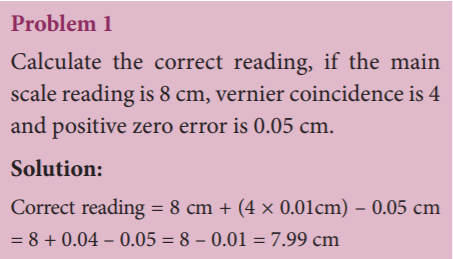30. The main scale reading is 8 cm and vernier coincidence is 4 and negative zero error is 0.02 cm.

1. 8 cm
2. 8.6 cm
3. 8.06 cm
4. 8.00 cm

Explanation31. Which of the following can be measured using Vernier Caliper?

1. Length
2. Width
3. Height
4. 1, 2
5. 1, 3
6. 2, 3
7. All the above

Explanation

We can use Vernier caliper to find different dimensions of any familiar object. If the length, width and height of the object can be measured, volume can be calculated. For example, if we could measure the inner diameter of a beaker (using appropriate jaws) as well as its depth (using the depth probe) we can calculate its inner volume.

32. Which of the following statement is correct?

1. Screw gauge is an instrument that can measure the dimensions up to 1/10th of a millimetre
2. With the screw gauge it is possible to measure the diameter of a thin wire and thickness of thin metallic plates
3. 1 alone
4. 2 alone
5. 1, 2
6. None

Explanation

Screw gauge is an instrument that can measure the dimensions up to 1/100th of a millimetre or 0.01 mm. With the screw gauge it is possible to measure the diameter of a thin wire and thickness of thin metallic plates.

33. The head of the sleeve (Thimble) is divided into____ divisions

1. 10
2. 100
3. 1000
4. 50

Explanation

The screw gauge consists of a U-shaped metal frame. A hollow cylinder is attached to one end of the frame. Grooves are cut on the inner surface of the cylinder through which a screw passes. On the cylinder parallel to the axis of the screw there is a scale which is graduated in millimetre. It is called Pitch Scale (PS). One end of the screw is attached to a sleeve. The head of the sleeve (Thimble) is divided into 100 divisions and it is called the Head scale

34. Which of the following statement is correct?

1. The screw gauge works on the principal that when a screw rotates in a nut
2. The distance moved by the tip of the screw is directly proportional to the number of rotations.
3. The pitch of the screw is the distance moved by the tip of the screw for one complete rotation of the head. It is equal to 1 mm in typical screw gauges.
4. 1, 2
5. 1, 3
6. 2, 3
7. All the above

Explanation

The screw gauge works on the principal that when a screw rotates in a nut, the distance moved by the tip of the screw is directly proportional to the number of rotations. The pitch of the screw is the distance moved by the tip of the screw for one complete rotation of the head. It is equal to 1 mm in typical screw gauges.

Pitch of the screw = Distance moved by the Pitch/ No. of rotations by Head scale

35. Match the following:

1. Gold 1. Tonnes
2. Medicines 2. Gram
3. Provisions 3. Milligram
4. Express cargo 4. Kg
5. 2, 1, 3, 4
6. 4, 1, 2, 3
7. 2, 3, 4, 1
8. 3, 1, 2, 4

Explanation

We commonly use the term ‘weight’ which is actually the ‘mass’. Many things are measured in terms of ‘mass’ in the commercial world. The SI unit of mass is kilogram (kg). In any case, the units are based on the items purchased. For example, we buy gold in gram or milligram, medicines in milligram, provisions in gram and kilogram and express cargo in tonnes.

36. The shell of an egg is___ % of its mass

1. 30
2. 20
3. 12
4. 35

Explanation

The shell of an egg is 12% of its mass. A blue whale can weigh as much as 30 elephants and it is as long as 3 large tour buses.

37. Which of the following is not standard reference mass of common beam-balance?

1. 10g
2. 20g
3. 25g
4. 50g

Explanation

A beam balance compares the sample mass with a standard reference mass (Standard reference masses are 5g, 10g, 20g, 50g, 100g, 200g, 500g, 1kg, 2kg, 5kg). This balance can measure mass accurately up to 5g

38. What are the standard reference masses used in this physical balance?

1. 10 mg
2. 100 mg
3. 1000 g
4. 1, 2
5. 1, 3
6. 2, 3
7. All the above

Explanation

Physical balance is used in labs and is similar to the beam balance but it is a lot more sensitive and can measure mass of an object correct to a milligram. The standard reference masses used in this physical balance are 10 mg, 20 mg, 50 mg, 100 mg, 200 mg, 500 mg, 1 g, 2g, 5 g, 10 g, 20 g, 50 g, 100g, and 200 g.

39. Spring balance works on the principle of___

1. Wuhar’s law
2. Hooke’s law
3. Newton’s 3rd law
4. Newton’s 1st law

Explanation

Spring balance helps us to find the weight of an object. It consists of a spring fixed at one end and a hook attached to a rod at the other end. It works by ‘Hooke’s law’ which states that the addition of weight produces a proportional increase in the length of the spring. A pointer is attached to the rod which slides over a graduated scale on the right. The spring extends according to the weight attached to the hook and the pointer reads the weight of the object on the scale.

40. Which of the following statement is incorrect?

1. Mass (m) is the force (N) exerted by the surface on the body to balance against gravitational pull on the object
2. Weight (w) is the quantity of matter contained in a body.
3. The gravitational force acting on the object is given by ‘mg’
4. 1, 2
5. 1, 3
6. 2, 3
7. All the above

Explanation

Mass (m) is the quantity of matter contained in a body. Weight (w) is the normal force (N) exerted by the surface on the body to balance against gravitational pull on the object. In the case of spring scale, the tension in the spring balances the gravitational pull on the object. When a man is standing on the surface of the earth or floor, the surface exerts a normal force on the body which is equivalent to gravitational force. The gravitational force acting on the object is given by ‘mg’. Here, m is mass of the object and ‘g’ is acceleration due to gravity.

41. If a man has a mass 50 kg on the earth, then what is his weight?

1. 500 N
2. 410 N
3. 490 N
4. 510 N

Explanation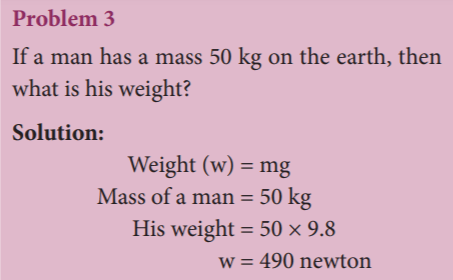42. Which of the following statement is correct?

1. The pull of gravity on the Moon is 1/6 times weaker than that on the Earth.
2. The weight of the object on the Moon to be less than that on the Earth by six times
3. Acceleration due to gravity on the Moon = 1.63 ms^–2
4. 1, 2
5. 1, 3
6. 2, 3
7. All the above

Explanation

The pull of gravity on the Moon is 1/6 times weaker than that on the Earth. This causes the weight of the object on the Moon to be less than that on the Earth by six times. Acceleration due to gravity on the Moon = 1.63 ms^–2 If the mass of a man is 70 kg then his weight on the Earth is 686 N and on the Moon is 114 N. But his mass is still 70 kg on the Moon.

43. Which of the following statement is correct?

1. Mass is a derived quantity
2. It is measured using physical balance.
3. Remains the same everywhere
4. 1, 2
5. 1, 3
6. 2, 3
7. All the above

Explanation